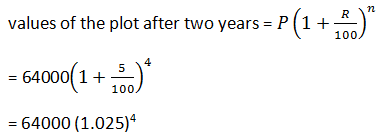# Exercise  14.5

### 1. Ms. Cherian purchases a boat for Rs. 16000. If the total cost of the boat is depreciating at the rate of 5% per annum, calculate its value after 2 years.

#### Solution:

Given,

Price of boat = Rs. 16000

Depreciation rate = 5% per annum

So,### 2. The value of a machine depreciates at the rate of 10% per annum. What will be its value 2 years hence, if the present value is Rs 100000? Also, find the total depreciation during this period.

#### Solution:= 100000 (0.90)2

= 81,000

Then, the value of the machine after two years will be Rs 81,000.

Depreciation = Rs 1000,000 – Rs 81,000

= Rs 19,000

### 3. Pritam bought a plot of land for Rs 640000. Its value is increasing by 5% of its previous value after every six months. What will be the value of the plot after 2 years?

#### Solution:

Given:

P: Rs 64,000

R = 5% for every sis months= Thus the value of the plot after two years will be Rs 706,440.25.

### 4. Three years ago, the population of a town was 50000. If the annual increase during three successive years be at the rate of 4%, 5% and 3% respectively, find the present population.

#### Solution:

According to question,

Population of town 3 years ago was = 50000

Annual increasing in 3 years = 4% ,5%, 3% respectively

Let present population = X

So,Then, Present population of town = 56238

### 5. The value of a machine depreciates at the rate of 10% per annum. It was purchased 3 years ago. If its present value is Rs 43740, find its purchase price.

#### Solution:Then, the purchase price of the machine was Rs 60,000.

### 6. The value of a refrigerator which was purchased 2 years ago, depreciates at 12% per annum. If its present value is Rs 9680, for how much was it purchased?

#### Solution:### 7. The cost of a T.V. set was quoted Rs 17000 at the beginning of 1999. In the beginning of 2000 the price was hiked by 5%. Because of decrease in demand the cost was reduced by 4% in the beginning of 2001. What was the cost of the T.V. set in 2001?

#### Solution:Then, the cost of the TV in 2001 was Rs. 17,136.

### Solution:Updated: December 3, 2019 — 3:30 pm